# CSS Lists

The List in CSS specifies the listing of the contents or items in a particular manner i.e., it can either be organized orderly or unorder way, which helps to make a clean webpage. It can be used to arrange the huge with a variety of content as they are flexible and easy to manage. The default style for the list is borderless. The list can be categorized into 2 types:

• Unordered List: In unordered lists, the list items are marked with bullets i.e. small black circles by default.
• Ordered List: In ordered lists, the list items are marked with numbers and an alphabet.

We have the following CSS lists properties, which can be used to control the CSS lists:

• list-style-type: This property is used to specify the appearance (such as disc, character, or custom counter style) of the list item marker.
• list-style-image: This property is used to set the images that will be used as the list item marker.
• list-style-position: It specifies the position of the marker box with respect to the principal block box.
• list-style: This property is used to set the list style.

List Item Marker

This property specifies the type of item marker i.e. unordered list or ordered. The list-style-type property specifies the appearance of the list item marker (such as a disc, character, or custom counter style) of a list item element. Its default value is a disc.

Syntax:

`list-style-type:value;`

The following value can be used:

• circle
• decimal, eg :1,2,3, etc
• lower-roman
• upper-roman
• lower-alpha, eg: a,b,c, etc
• upper-alpha, eg: A, B, C, etc
• square

Example: This example describes the CSS List with the various list-style-type where the values are set to square & lower-alpha.

## HTML

 `` `<``html``>` `  `  `<``head``>` `    ``<``style``>` `        ``ul.a {` `            ``list-style-type: square;` `        ``}`   `        ``ol.c {` `            ``list-style-type: lower-alpha;` `        ``}` `    ``` ``   `<``body``>` `    ``<``h2``>` `        ``GeeksforGeeks` `    ``` `    ``<``p``> Unordered lists ` `    ``<``ul` `class``=``"a"``>` `        ``<``li``>one` `        ``<``li``>two` `        ``<``li``>three` `    ``` `    ``<``ul` `class``=``"b"``>` `        ``<``li``>one` `        ``<``li``>two` `        ``<``li``>three` `    ``` `    ``<``p``> Ordered Lists ` `    ``<``ol` `class``=``"c"``>` `        ``<``li``>one` `        ``<``li``>two` `        ``<``li``>three` `    ``` `    ``<``ol` `class``=``"d"``>` `        ``<``li``>one` `        ``<``li``>two` `        ``<``li``>three` `    ``` `` `  `  ``

Output: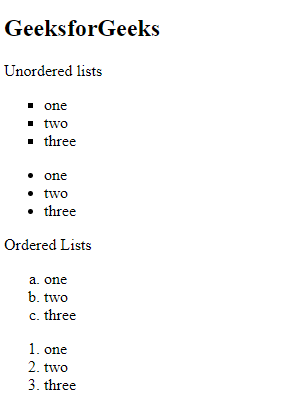Image as List Marker: This property specifies the image as a list item marker. The list-style-image property is used to sets an image to be used as the list item marker. Its default value is “none”.

Syntax:

`list-style-image: url;`

Example: This example describes the CSS List with the various list-style-image where the values are set to url of the image.

## HTML

 `` `<``html``>` `  `  `<``head``>` `    ``<``title``> CSS list-style-image Property ` `    ``<``style``>` `        ``ul {` `            ``list-style-image: ` `url("https://contribute.geeksforgeeks.org/wp-content/uploads/listitem-1.png");` `        ``}` `    ``` ``   `<``body``>` `    ``<``h1``>` `        ``GeeksforGeeks` `    ``` `    ``<``p``> Unordered lists ` `    ``<``ul``>` `        ``<``li``>1` `        ``<``li``>2` `        ``<``li``>3` `    ``` `` `  `  ``

Output: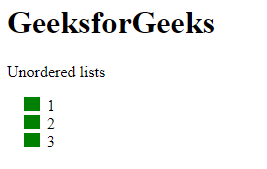List Marker Position: This property specifies the position of the list item marker. The list-style-position property is used to sets the position of the marker relative to a list item. Its default value is “outside”.

There are 2 types of position markers:

• list-style-position: outside; In this, the bullet points will be outside the list item. The start of each line of the list will be aligned vertically.

Syntax:

`list-style-position: outside;`

Example: This example describes the CSS List with the various list-style-position where the value is set to outside.

## HTML

 `` `<``html``>` `  `  `<``head``>` `    ``<``style``>` `        ``ul.a {` `            ``list-style-position: outside;` `        ``}` `    ``` ``   `<``body``>` `    ``<``h2``>` `        ``GeeksforGeeks` `    ``` `    ``<``p``> Unordered lists ` `    ``<``ul` `class``=``"a"``>` `        ``<``li``>one` `            ``<``br``>` `            ``In this the bullet points will be ` `            ``outside the list item.` `        ``` `        ``<``li``>two` `            ``<``br``>` `            ``The start of each line of the list ` `            ``will be aligned vertically.` `        ``` `        ``<``li``>three` `    ``` `` `  `  ``

Output: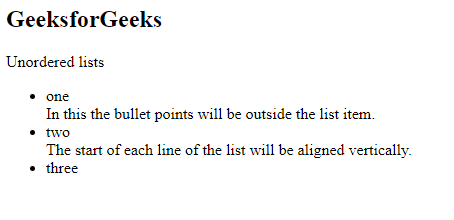• list-style-position: inside; In this, the bullet points will be inside the list. The line along with the bullet points will be aligned vertically.

Syntax:

`list-style-position: inside;`

Example: This example describes the CSS List with the various list-style-position where the value is set to inside.

## HTML

 `` `<``html``>` `  `  `<``head``>` `    ``<``style``>` `        ``ul.a {` `            ``list-style-position: inside;` `        ``}` `    ``` ``   `<``body``>` `    ``<``h2``>` `        ``GeeksforGeeks` `    ``` `    ``<``p``> Unordered lists ` `    ``<``ul` `class``=``"a"``>` `        ``<``li``>one` `            ``<``br``>` `            ``In this the bullet points will ` `            ``be inside the list item.` `        ``` `        ``<``li``>two` `            ``<``br``>` `            ``The line along with the bullet points ` `            ``will be aligned vertically..` `        ``` `        ``<``li``>three` `    ``` `` `  `  ``

Output: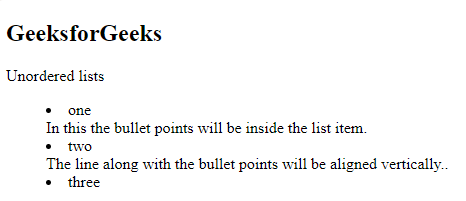Shorthand Property: Shorthand Property allows us to set all the list properties in one command. The order of property is a type, position, and image. If any of the properties is missing, the default value is inserted.

Example: This example describes the CSS List using the shorthand property.

## HTML

 `` `<``html``>` `  `  `<``head``>` `    ``<``style``>` `        ``ul.a {` `            ``list-style: square inside;` `        ``}` `    ``` ``   `<``body``>` `    ``<``h2``>` `        ``GeeksforGeeks` `    ``` `    ``<``p``> Unordered lists ` `    ``<``ul` `class``=``"a"``>` `        ``<``li``>one` `        ``<``li``>two` `        ``<``li``>three` `    ``` `` `  `  ``

Output: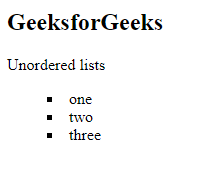Styling Lists: The list can be formatted in CSS. Different colors, borders, backgrounds, and paddings can be set for the lists.

Example: This example describes the CSS List where the various styling properties are applied to the element.

## HTML

 `` `<``html``>` `  `  `<``head``>` `    ``<``style``>` `        ``ul.a {` `            ``list-style: square;` `            ``background: pink;` `            ``padding: 20px;` `        ``}` `    ``` ``   `<``body``>` `    ``<``h2``>` `        ``GeeksforGeeks` `    ``` `    ``<``p``> Unordered lists ` `    ``<``ul` `class``=``"a"``>` `        ``<``li``>one` `        ``<``li``>two` `        ``<``li``>three` `    ``` `` `  `  ``

Output: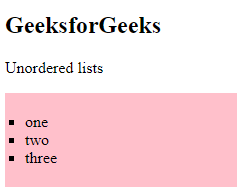Nested List: Lists can also be nested. We have sub-sections for sections, so we need the nesting of lists.

Example: This example describes the CSS List having a list declared inside another list.

## HTML

 `` `<``html``>` `  `  `<``body``>` `    ``<``h2``>` `        ``GeeksforGeeks` `    ``` `    ``<``ul``>` `        ``<``li``>` `            ``one` `            ``<``ul``>` `                ``<``li``>sub one` `                ``<``li``>sub two` `            ``` `        ``` `        ``<``li``>` `            ``two` `            ``<``ul``>` `                ``<``li``>sub one` `                ``<``li``>sub two` `            ``` `        ``` `        ``<``li``>` `            ``three` `            ``<``ul``>` `                ``<``li``>sub one` `                ``<``li``>sub two` `            ``` `        ``` `    ``` `` `  `  ``

Output: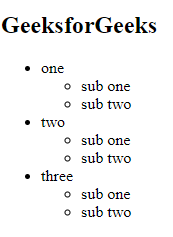Supported Browsers: